# Make an MPPT Solar charge Controller with Synchronous Buck Converter

MPPT Solar charge controller is one of the most useful devices in solar power system. In this project, we are going to learn to make our own MPPT solar charge controller. Here we will use a synchronous buck converter and as a side option, we’ll keep Bluetooth datalogger and cell phone charge with this device. Keep reading…

Disclaimer: Electricity is always dangerous. Skill required to work with electricity. Do work with your own risk. Author will not be responsible for any misuse or harmful act. This website contents is also copyright protected. Anything copied and directly posted in your website and claiming it as yours is prohibited and nonsensical. Author published all the articles as opensource to help you making your project and learning purpose only. Learn and make one for yourself. If need any help feel free to ask the author. Author will be helpful to you. Thanks.

Table of Contents

## What is Solar Power System?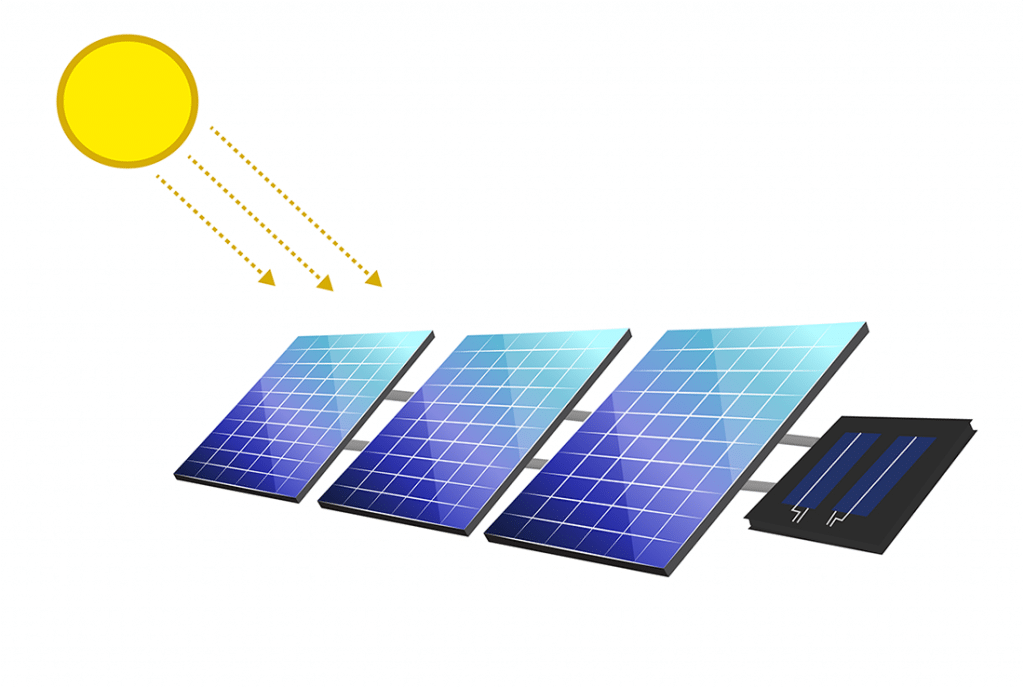Solar panel converting sunlight into electricity

Before everything we have to know what is solar home system or what is solar power system. Solar energy comes from Sun. The sunlight is converted into electricity with the help of Solar panel which is made of Semi-conductors (P-N junction).

This electricity is DC and can be stored or used to drive appliances like lights, fans etc. But as the power is DC we may need to convert it into AC to run our standard AC appliances at home. Anyway, the total system is consists of Solar panel, Battery as storage, Inverters if required and a charge controller.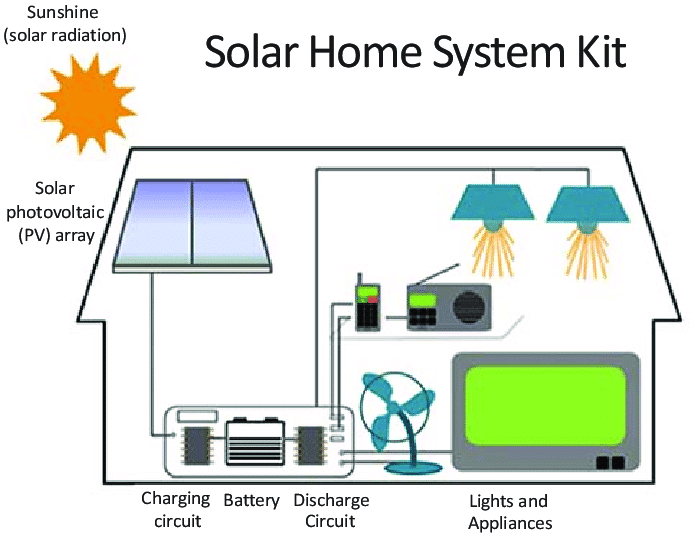Solar Home system

### What is Storage(Battery) in solar home system?

Before we start with charge controller, we need to know what is the storage system of a solar home system. In solar home system or solar power system, different types of batteries are used as the storage device. Most of the system use common Lead-Acid battery. But AGM and Lithium Ion batteries are also popular in solar power system. Among these three types Li-ion is the best but costly as well. That is why, Lead-Acid batteries are use in most of the small solar system.

Now, whatever the battery is each battery have specific charging and discharging characteristics. Each battery have a higher charging point above which battery should not be charged and have a lower discharging point below which point no battery should not be discharged any more.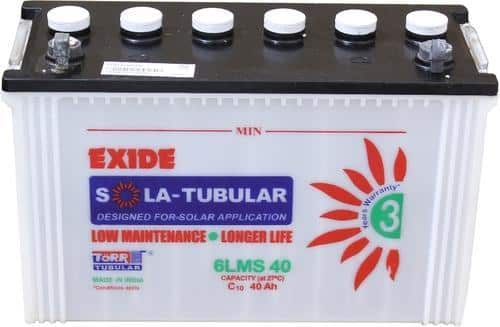A solar lead acid battery

If we consider a lead acid battery of 12V, a higher cut-off point will be 14.3V-14.6V (varies by different manufacturer). And a lower discharging point will be 11.5V-11.6V. That means, this battery should not be charged over 14.6V and should not be discharged below 11.5V.

That is why a charge controller is required in between the Solar panel and the battery.

### What is solar panel?

The term solar panel is used colloquially for a photo-voltaic (PV) module.

A PV module is an assembly of photo-voltaic cells mounted in a frame work for installation. Photo-voltaic cells use sunlight as a source of energy and generate direct current electricity. A collection of PV modules is called a PV Panel, and a system of Panels is an Array. Arrays of a photovoltaic system supply solar electricity to electrical equipment. Know more about solar panel from wiki.

Now lets see what is solar charge controllers. There are 3 types of charge controller available in the market. Lets check.

### What is solar charge controller?

A solar charge controller is that device which controls the charging and some of them also controls discharging of the battery. Normally it is consists of a switch between solar panel and battery. Controlling this switch, charging is regulated.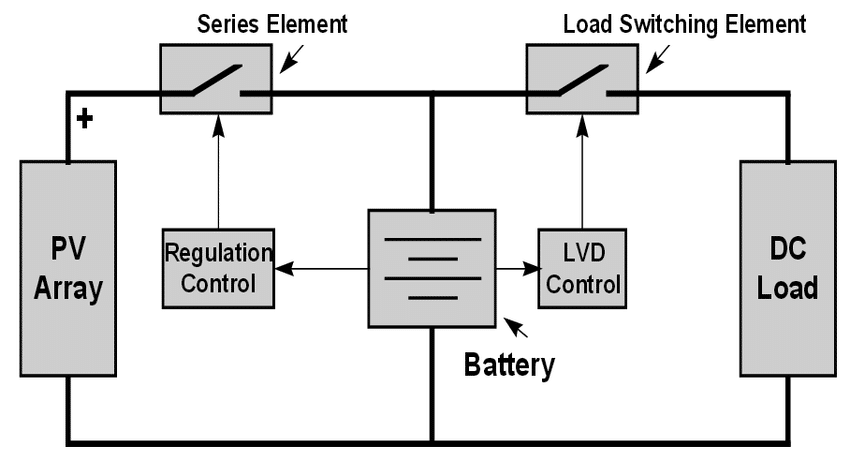Charge controller basic block diagram

Depending on charging mechanism, charge controllers can be differentiated into 3 types.

1. On/Off type charge controller
2. PWM type charge controller
3. MPPT type charge controller

### What is On/Off type charge controller?

A on/off type charge controller is the most basic one which have only a on/off switch like a MOSFET/Transistor/even relay and a controller (Analog circuit / micro-controller).

pros:On/Off type solar charge controller
1. Very easy circuit diagram
2. Simple operation
3. Low cost

cons:

1. Very low efficiency
2. Less charging

Due to very low efficiency, on/off type charge controllers are used in very limited systems. Specially where efficiency is not a factor this type of charge controller is used. If a solar panel is of 12V, this panel generates an open circuit voltage of 22V. As a 12V system have a battery of 12V, a on/off type charge controller can connect the PV to battery almost directly. That means, 22-12 = 10V is not used. At the same time if the panel provides 5A of current, total loss will be 10×5 = 50Watt. Even if we consider the panel in its peak power point, at least (16.5V-12V)x5A = 22.5Watt loss of energy.

Due to this type of loss, On/off type solar charge controller is used in limited systems.

### What is PWM type solar charge controller?PWM type solar charge controller

PWM type is an advance version of on/off type solar charge controller. A pwm signal is used to control the switching rather than direct on/off. This type of charge controller is little better than on/off type charge controller but still can not use the maximum generated power.

A PWM charge controller have smoother control in battery charging. Which enlarge battery life.

pros:

1. Smoother charging control
2. Longer battery life
3. Low price

cons:

1. Low efficient than MPPT
2. Power loss

### What is MPPT solar charge controller?MPPT solar charge controller

A MPPT solar charge controller is one kind of DC/DC converter which can deliver the maximum power generated by the solar panel to battery to store the charge. It is the most complex one among solar charge controller.

A MPPT solar charge controller mostly have only charging part. That means, it only controls solar panel to battery charging. For load control, another device may required depending on power system.

pros:

1. Highly efficient charging
2. Best energy management
3. Proper utilization of battery charging

cons

1. High cost
2. Complex circuitry.

We have known types of charge controllers, now lets see more about MPPT solar charge controller.

## Why we need MPPT solar charge controller?

MPPT solar charge controller is necessary for any solar power systems need to extract maximum power from PV module; it forces PV module to operate at voltage close to maximum power point to draw maximum available power. MPPT solar charge controller reduces complexity of system while output of system is high efficiency.

Each solar panel (PV) produces its maximum power near about 17V (16.5V most of cases). This point is known as MPP or Maximum Power Point. So the duty of MPPT solar charge controller is to maintain PV voltage at this MPP so that the available maximum power can be harvested from that solar panel (PV).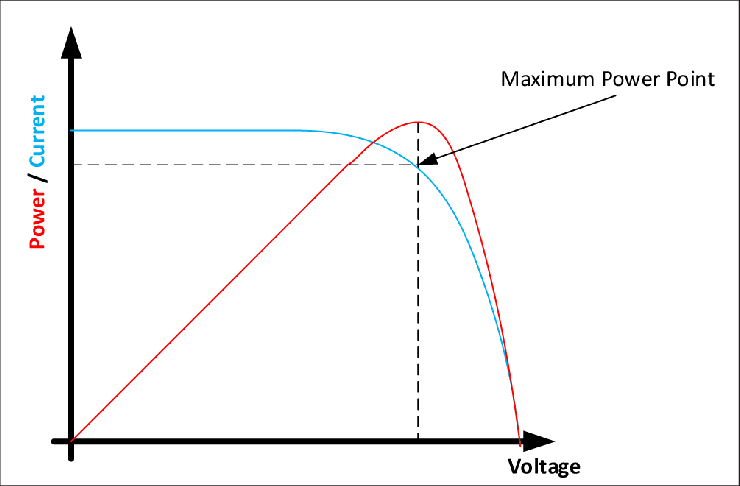Maximum power point

## MPPT charge Controller basic parts:

MPPT solar charge controller have a basic part DC/DC converter and a sensing part and a controller. The controller senses PV voltage and current and sends these data to the controller. The controller calculates the recent power, compare this power with previous power and decide what to do next. Changing control pulses, control maintain the DC/DC conversion in that point where the maximum power can be obtained on that time.

Now we have some basic parts in MPPT solar charge converter:

1. A DC/DC converter
2. Voltage and Current sensor and
3. A controller
4. Algorithm for MPPT.

Les’s see one by one next.

## What is a DC/DC converter?

A DC/DC converter is that converter where the output voltage can be lower or higher than the input voltage. Based on configuration, DC/DC converter can be dived into these types:

• Buck Converter
• Boost Converter
• Buck-Boost converter
• Ćuk Converter

### What is Buck converter?

Buck converter is a step down converter. Here Output voltage is lower than input voltage. A basic diagram of buck converter is:Buck converter (Asynchronous)

#### Types of Buck converter?

Based on switching configuration, buck converter can be divided into two types.

1. Synchronous Buck converter
2. Asynchronous Buck converter

We will discuss about Synchronous buck converter later.

### What is Boost converter?

Boost converter is one kind of step-up converter where output voltage is higher than input voltage. A basic diagram of boost converter is: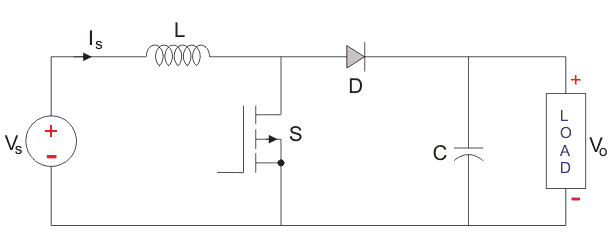Boost converter

### What is buck-boost converter?

Buck-Boost converter is a combined dc/dc converter. Here the output voltage can be higher or lower than the input voltage. By controlling two pwm the output can be controlled. The basic diagram for buck-boost converter is: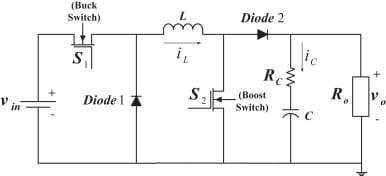Buck-Boost converter

### What is Ćuk Converter?

The Ćuk converter is a type of DC/DC converter that has an output voltage magnitude that is either greater than or less than the input voltage magnitude. It is essentially a boost converter followed by a buck converter with a capacitor to couple the energy.Ćuk converter

### What is Synchronous Buck Converter?Asynchronous buck converter

In Asynchronous buck converter, there is a diode connected inversely to wheel the current through inductor. But as there is a forward voltage drop in diode of 0.7V, a loss happen here. This loss decreases the total efficiency of a buck converter. If this diode is replaced by a MOSFET, the forward voltage drop can be reduced to 0.1V. Which increases total converter efficiency.

The synchronous buck converter is used to step a voltage down from a higher voltage to a lower voltage. Synchronous buck converters are very popular in industry today and provide high efficiency solutions for a wide
range of applications. This application note gives the formulas to calculate the power stage of a synchronous buck operating in continuous conduction mode. A synchronous buck converter produces a regulated voltage that is lower than its input voltage and can deliver high current while minimizing power loss. As shown in Figure 1, the synchronous buck converter is comprised of two power MOSFETs, an output inductor, and input and output capacitors.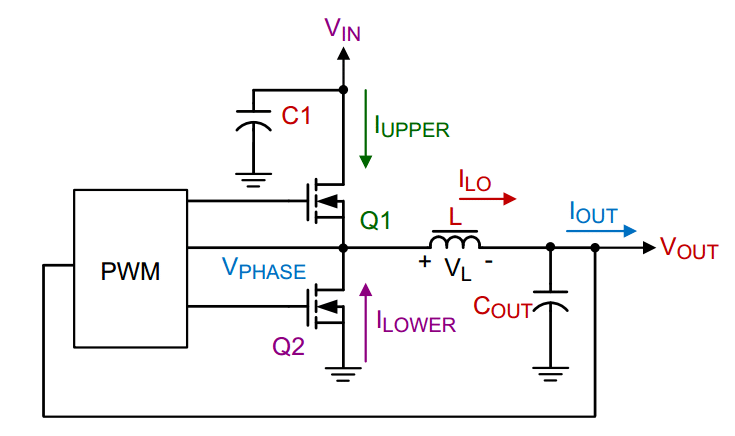Figure1: Basics of a synchronous Buck converter

Q1, the high side MOSFET, is connected directly to the input voltage of the circuit. When Q1 turns on, IUPPER is supplied to the load through Q1. During this time the current through the inductor increases (charging L) and Q2 is off. When Q1 turns off, Q2 turns on and ILOWER is supplied to the load through Q2. During this time, the inductor current decreases (discharging L). Figure 2 shows the basic waveforms for the synchronous buck converter in continuous conduction mode.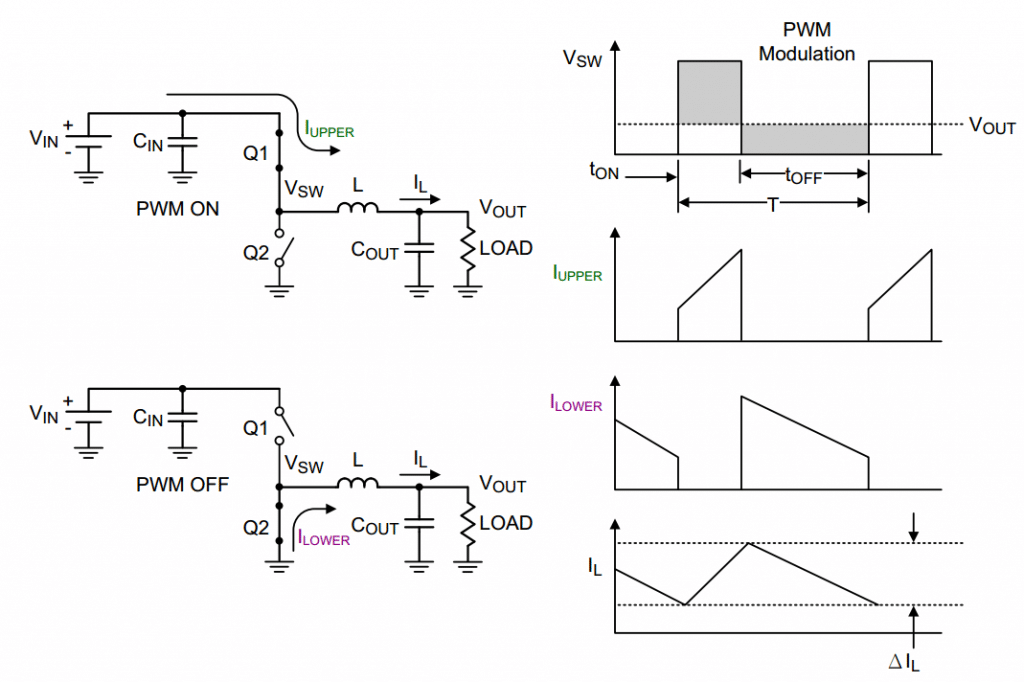Synchronous buck converter waveform

To learn more about Synchronous buck converter design please read these documents:

Download Application Notes for buck converter design guide.

### Inductor design guide:

Inductor is the most important part of a DC/DC converter. Selecting and designing an inductor is little bit complex too. Here in our project, toroidal code inductor is used. To calculate the inductor, I took help from coil32.net. You can check that online tool, it is pretty helpful. After making your inductor, you should check the inductance by a RLC meter. I had this meter, and I checked the inductance with it before circuit assembly.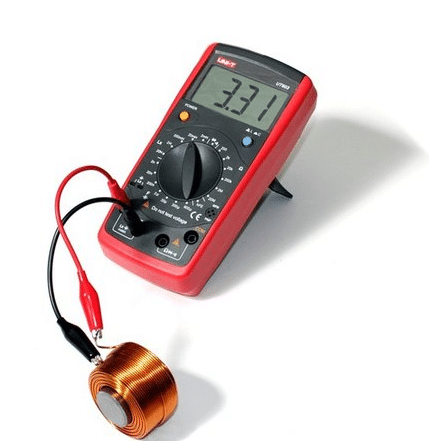RLC meter

## Voltage and current sensing unit:

Voltage can be sensed easily using resistor voltage divider and capacitor filter like this circuit: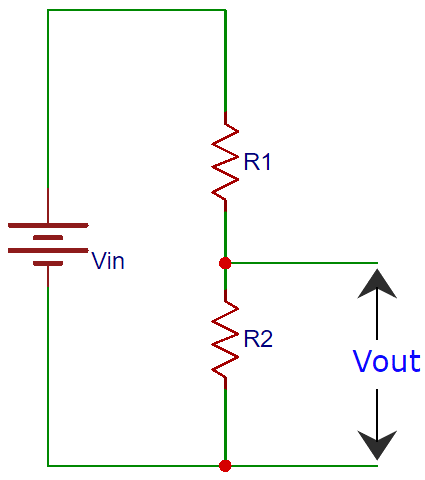Resistor voltage divider circuit to sense voltage

But to sense current, using hall-effect sensor is more efficient way than using series resistor. Here in this project, we’ll use ACS712-30 hall-effect based current sensor.ACS712 Current sensor

This current sensor provides linear dc voltage corresponding to the current flow. Neutral point if 2.5V (where supply voltage is 5V). Positive or negative current flow generate a linear voltage at the output terminal either higher than 2.5V or lower than 2.5V correspondingly.

ACS712-30A gives 66mV/Amp deflection according to the input current flow. We can easily integrate this current sensor in our circuit as the current sensor.

## About the controller:

As the controller, we can use micro-controller. Or we can say that we have to use a micro-controller to control everything. Here in this project, we will use PIC16F877A micro-controller.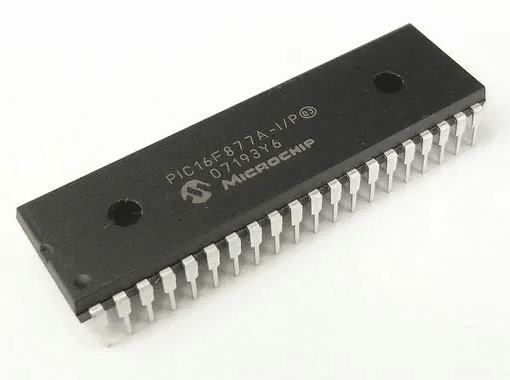PIC16F877A as out controller

## Algorithm:

For Maximum Power Point Tracking, an algorithm is very important. Depending on algorithm, the total design can be changed. For MPPT charge controllers, There are most common algorithms listed below:

1. Perturbation and Observation (P&O)
2. Incremental Conductance (IncCond)
3. Ripple Correlation
4. Constant Voltage
5. Open-Circuit
6. Current sweep
7. Temperature Method
8. Fractional open-circuit voltage

Discussing individually will be too lengthy. I’m requesting you to study on this yourself.

## Other parts of MPPT solar charge controller:

Beside the main parts of MPPT solar charge controller, we also need to focus on other associated parts for our Project.

1. Input and output protections
2. Gate driver for MOSFETs
3. Visualization of information
4. Blue-tooth data logging
5. SMPS mobile charging
6. Power supply for circuit parts.
7. Load control unit

### Input and Output protection:

Input and output should be protected from reverse flow and a current limiting must be introduced.

For reverse flow, a Diode can be used. But as efficiency is an issue, we have to eliminate this diode by a MOSFET. The MOSFET also have a body diode. If we use the MOSFET in reverse way, the body diode will work as normal diode and while switching the upper MOSFET of the Synchronous buck converter, if we switch this MOSFET, it will short the diode. That means, the voltage drop will be less than 0.1V. Thus, we can reduce loss as well as can use a diode in efficient way. This configuration in our project will be: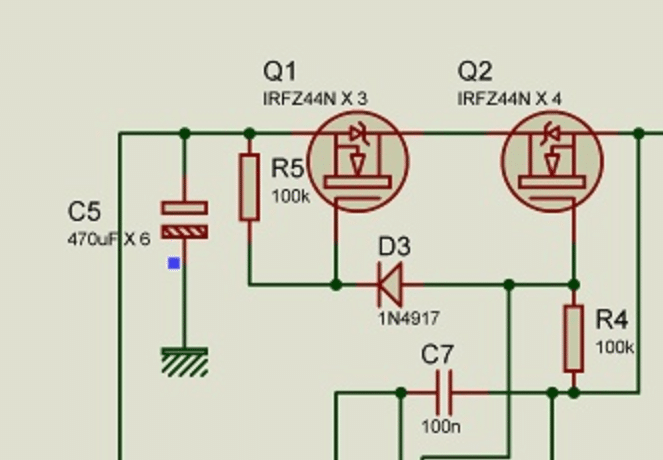MOSFET as diode

For current limit, we can use Fuses. Using DC fuse is good idea but if there is no DC fuse nearby, we can use common fuses.Fuse

### Gate Driver circuit: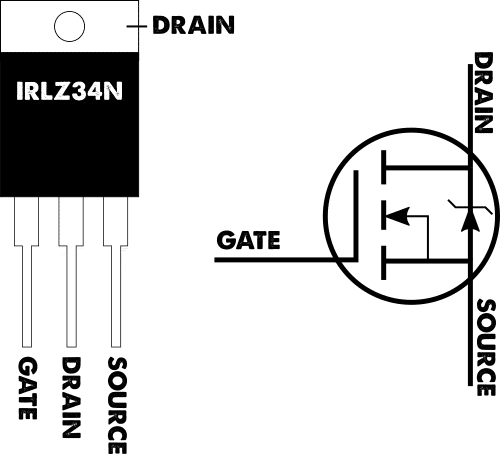MOSFET configuration

Most of the micro-controllers work at 5V supply and others are 3.3V or even 2.5V range. But the MOSFETs need at least 10V across its Gate and Source for switching. Otherwise conductivity will be low which generates heat and in the end, MOSFET blows out. As our micro-controller can not provide more than 5V so to switch a MOSFET we must need a gate driving circuit. This circuit can be designed in multiple way depending on circuit configuration.

Here in this project, the DC/DC converter is Synchronous Buck type. In synchronous buck converter, we already know that there are two MOSFETs. One is upper MOSFET and other one is lower MOSFET.Gate driving circuit

The gate signal of these two MOSFETs are inverted to each other type like this: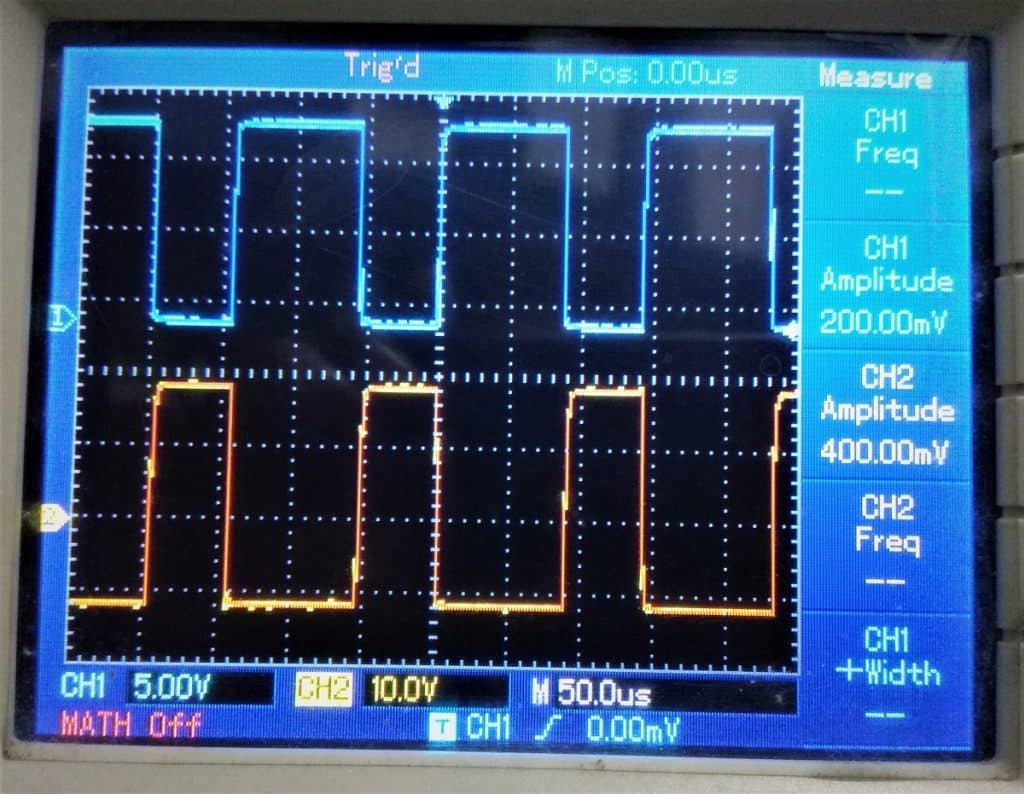MOSFET Gate driving signal

Here the blue one on the top is the gate driving signal of upper MOSFET and the red one on the bottom is the gate driving signal for the lower MOSFET. In this way, the back emf of inductor can be pass through the lower MOSFET which was generated by the upper MOSFET switching. This way the synchronous buck converter works.

#### Why we need a Gate Driving IC?

As you see, the gate driving mechanism in Synchronous buck converter is little bit complex. A dedicated gate driving IC can this job efficiently. That is why, we need a gate driving IC.

Here IR2104 is one of the suitable gate driving IC for Synchronous buck converter.

#### About IR2104 gate driving IC: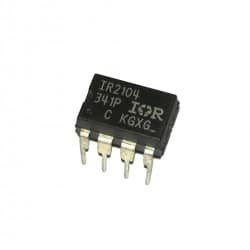IR2104 Gate driver IC

IR2104 is dedicated gate driving IC for half bridge driver which is actually a kind of Synchronous buck converter gate driver IC.

Check the datasheet of IR2104 here to understand about this gate driver IC.

The IR2104(S) are high voltage, high speed power MOSFET and IGBT drivers with dependent high and low side referenced output channels. Proprietary HVIC and latch immune CMOS technologies enable ruggedized monolithic construction. The logic input is compatible with standard CMOS or LSTTL output, down to 3.3V logic. The output drivers feature a high pulse current buffer stage designed for minimum driver cross-conduction. The floating channel can be used to drive an N-channel power MOSFET or IGBT in the high side configuration which
operates from 10 to 600 volts.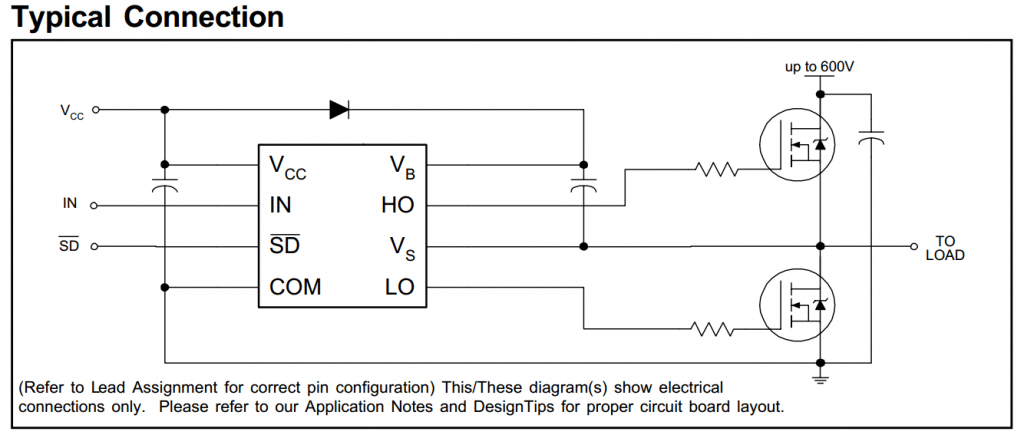Basic circuit for IR2104

In IR2104, the input signal is given to INPUT (Pin#2) of the IC. A active low signal at SD pin stops the output to drive. A bootstrap capacitor of 0.1uF works fine but this capacitor must be the best quality (low ESR).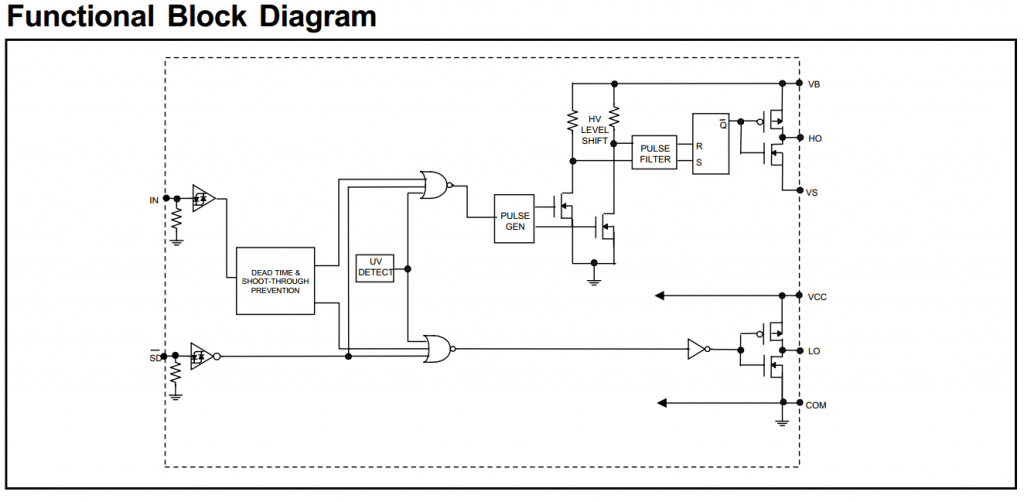Block diagram of IR2104

### Visualization of information: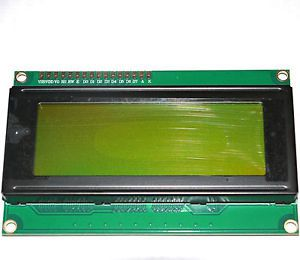20×4 LCD display

For visualization, a very common device is LCD display. In this project, we’ll used 20×4 LCD to visualize information. Besides, LEDs are used to indicate mode of operation.

This LCD need 6 pin for communication and the pin diagram of LCD is: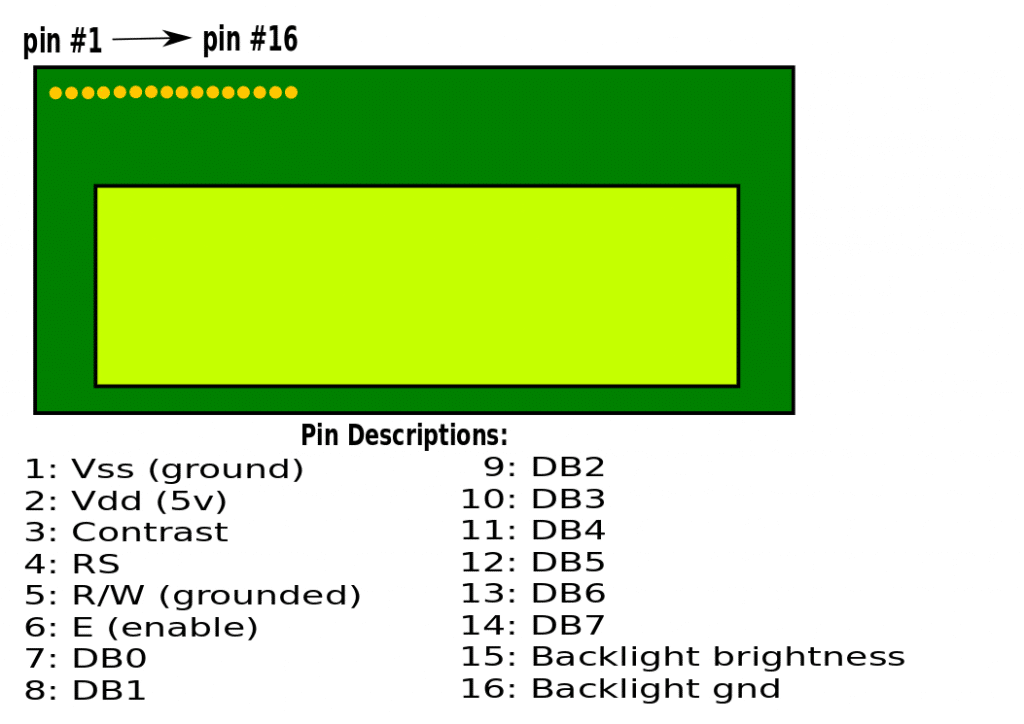LCD pin diagram

### Blue-tooth data logging:

For remote data logging, a blue-tooth module is used here. Although memory cards are used in most of the commercial device as field data logger, but here in this project we are not logging data locally. We kept an option to monitor the charge controller data remotely. That is why, a bluetooth module HC06 is used.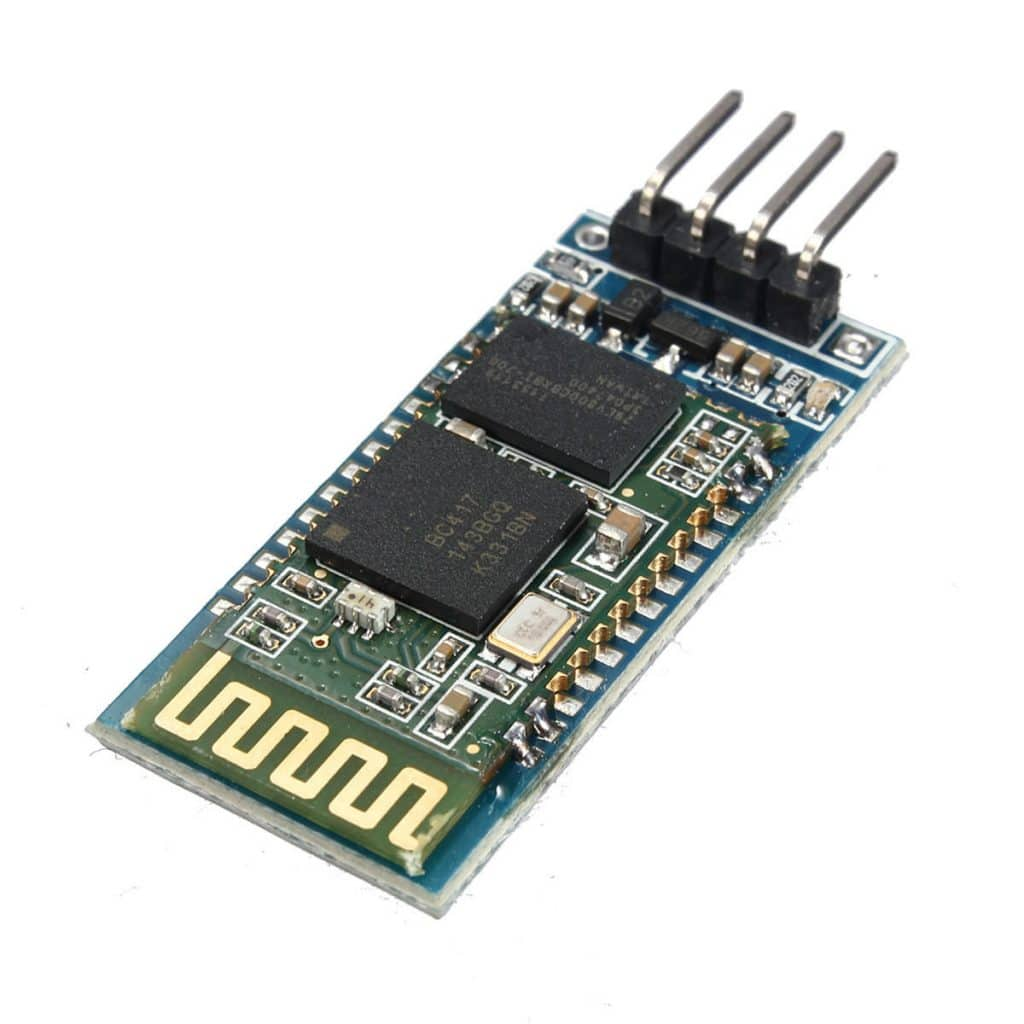HC06 Bluetooth module

This is a very common bluetooth module. Simple connection through UART module of micro-controller. Can be connect with android cell phone and an app on the phone can be used to monitor and log the data.

### SMPS mobile charging:

A mobile charge which was not so important part of a MPPT solar charge controller but kept in design to make the project more useful and interesting. Here, a Switch Mode Power Supply circuit is designed with MC34063A IC which can supply 5V at 350mA very easily. The circuit diagram for our mobile charger is: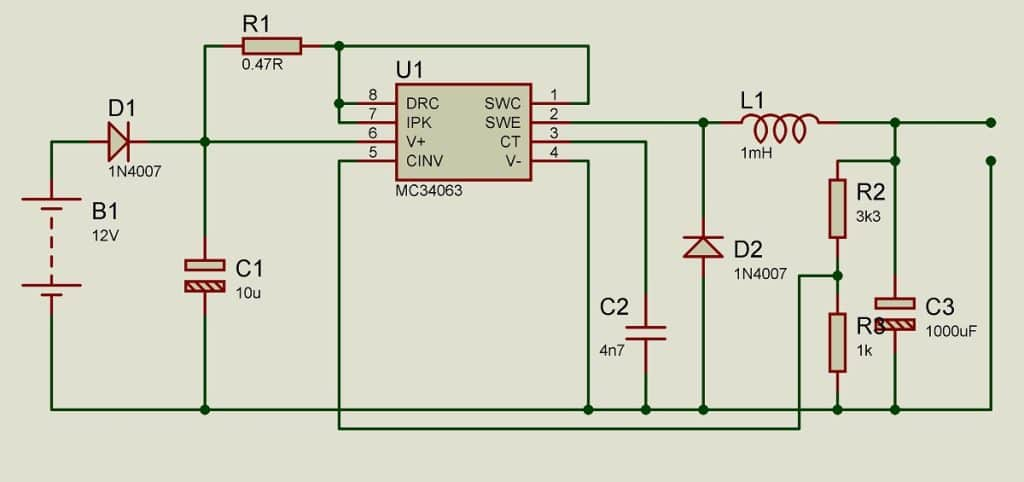Mobile charger circuit

You can download the datasheet for MC34063A from here.

### Power supply for circuit parts:

There are micro-controller, ACS current sensors, Bluetooth module, LCD display all these devices need power to run. All these components need 5V DC supply. This power supply is designed with LM7805 voltage regulator IC. The circuit diagram is: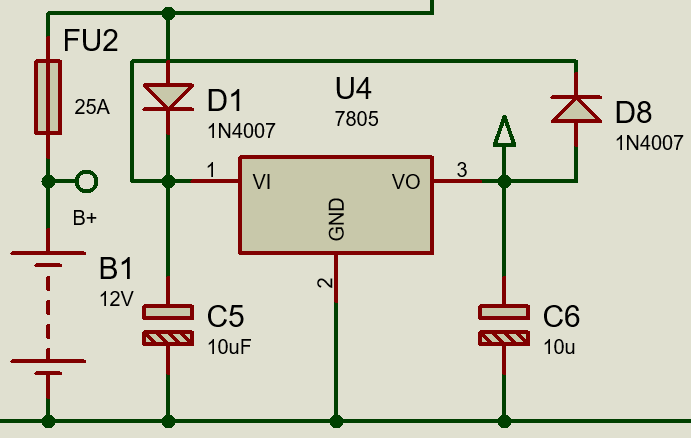5V Power supply

### Load control unit:

Although most of the MPPT solar charge controller doesn’t have load control unit, but in our project we kept this part. Loads are supplied from our battery and switched by a MOSFET. A gate driver (Opto-coupler: P817C) is introduced as well to drive the MOSFET gate.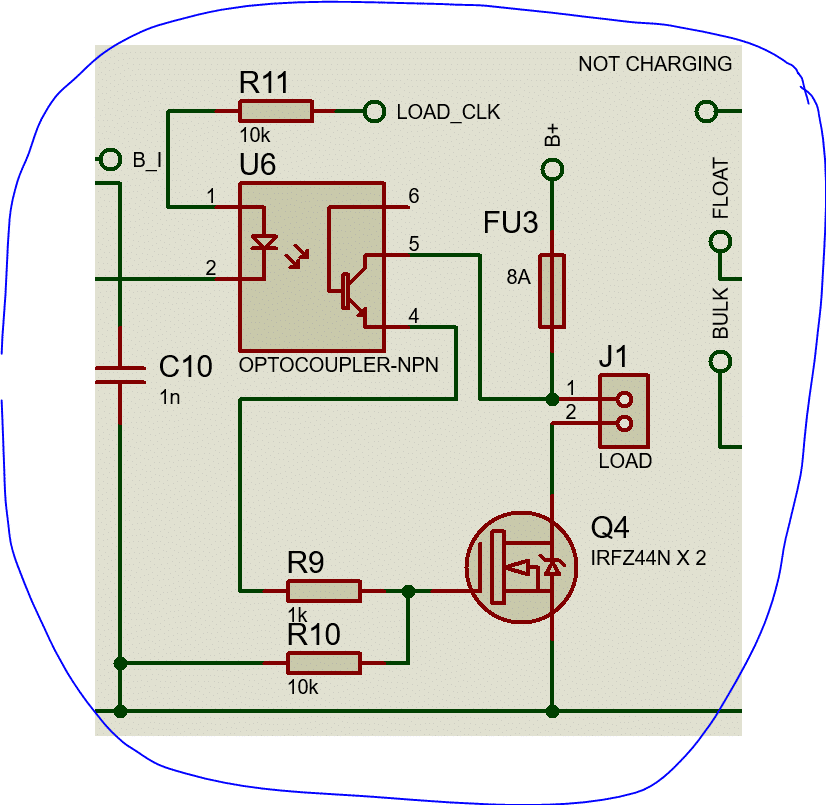Load control unit

## Combing all together:

So our MPPT solar charge controller has various parts which is working in combination. If we short list again, we can make this:

1. A DC/DC buck converter: Synchronous Buck Converter
2. Gate driving circuit: IR2104
3. Protecting Circuit: Fuse + Diode(MOSFET)
4. Load control circuit: MOSFET & Gate driver
5. Data-logging circuit: Blue-tooth Module (HC06)
6. Sensing circuit: ACS712-30 & resistors
7. Power supply circuit: LM7805 circuit
8. Mobile charge circuit: MC34063A circuit

## Block diagram:

Block Diagram of our project is: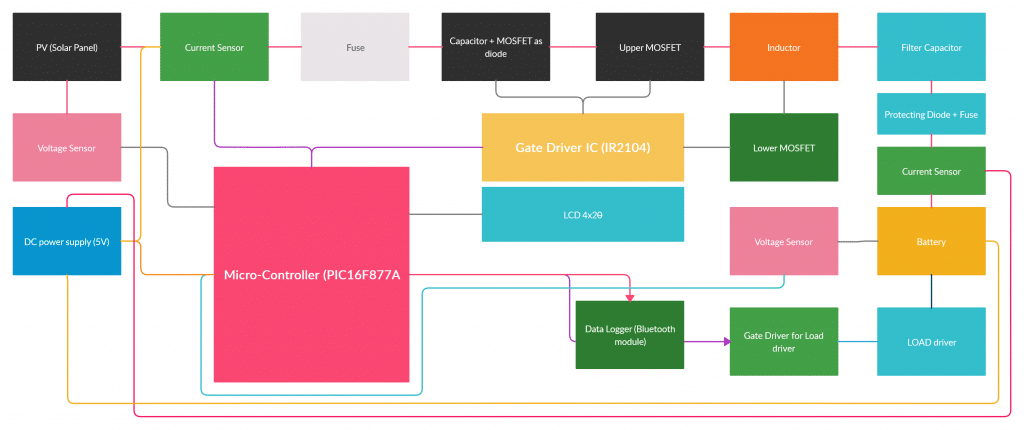Block Diagram

## Circuit Diagram:

Here is the circuit diagram of this project: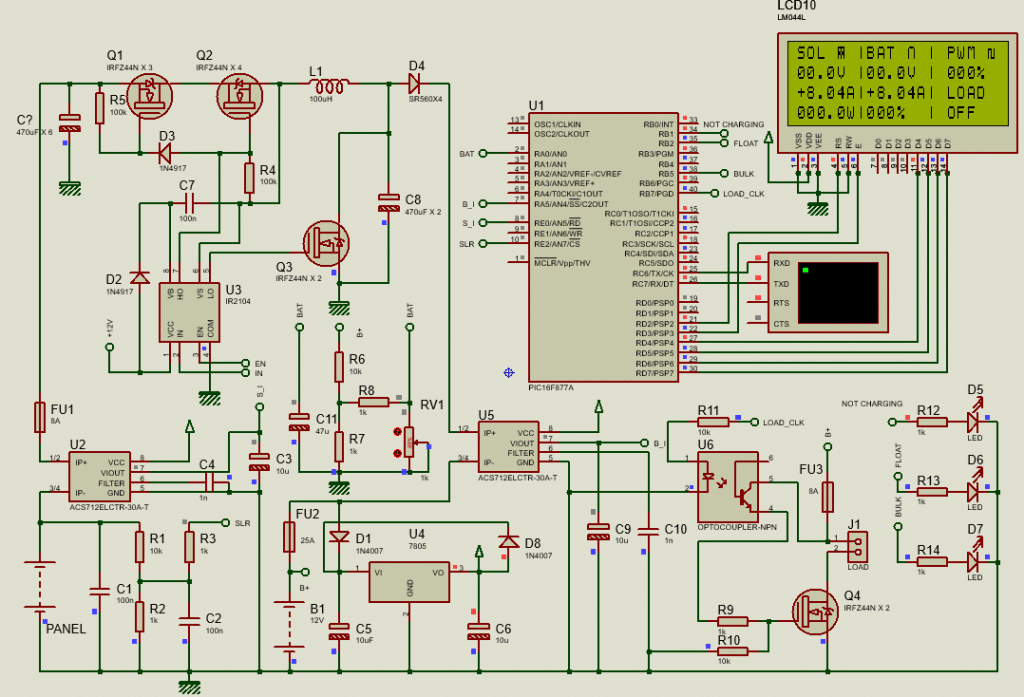Circuit diagram of MPPT solar charge controller

As this is a big power circuit, proteus is not suitable for simulation. Error result in simulation is very common in this type of big project.

You can download the proteus file from here.

## Simulation Result:Simulation result

## Coding:

I’ve bought mikroC license at 2014 as I work professionally. So all of my project based on PIC micro-controllers are programmed using this compiler. Here is the mikroC code for this project.

```/*******************************************************************************
*     Program for, "MPPT Solar Charge Controller using Sync. Buck converter"   *
*                  Program Written by_ Engr. Mithun K. Das                     *
*                         MCU: PIC16F877A; X-Tal:8MHz                          *
*                               Date:17-01-2016                                *
*******************************************************************************/
// LCD module connections
sbit LCD_RS at RD2_bit;
sbit LCD_EN at RD3_bit;
sbit LCD_D4 at RD4_bit;
sbit LCD_D5 at RD5_bit;
sbit LCD_D6 at RD6_bit;
sbit LCD_D7 at RD7_bit;

sbit LCD_RS_Direction at TRISD2_bit;
sbit LCD_EN_Direction at TRISD3_bit;
sbit LCD_D4_Direction at TRISD4_bit;
sbit LCD_D5_Direction at TRISD5_bit;
sbit LCD_D6_Direction at TRISD6_bit;
sbit LCD_D7_Direction at TRISD7_bit;
// End LCD module connections
void Lcd_COut(char row, char col, const char *cptr)
{
char chr = 0;             //first, it is used as empty string
Lcd_Out(row, col, &chr);  //nothing to write but set position.
for ( ; chr = *cptr ; ++cptr ) Lcd_Chr_CP(chr); //out in loop
asm CLRWDT;
}
void UART_Write_CText(const char *cptr)
{
char chr;
for ( ; chr = *cptr ; ++cptr ) UART1_Write(chr);
}
const char character6[] = {0,29,21,21,21,21,23,0};
const char character5[] = {31,21,21,27,27,21,21,31};
const char character4[] = {14,17,17,17,17,17,17,31};
const char character3[] = {14,17,17,17,17,17,31,31};
const char character2[] = {14,17,17,17,17,31,31,31};
const char character1[] = {14,17,17,31,31,31,31,31};
const char character[] = {14,31,31,31,31,31,31,31};
void CustomChar(char pos_row, char pos_char,char num)
{
char i;
switch(num)
{
case 0:
{
Lcd_Cmd(64);
for (i = 0; i<=7; i++)Lcd_Chr_CP(character[i]);
Lcd_Cmd(_LCD_RETURN_HOME);
Lcd_Chr(pos_row, pos_char, num);
}
case 1:
{
Lcd_Cmd(72);
for (i = 0; i<=7; i++)Lcd_Chr_CP(character1[i]);
Lcd_Cmd(_LCD_RETURN_HOME);
Lcd_Chr(pos_row, pos_char, num);
}
case 2:
{
Lcd_Cmd(80);
for (i = 0; i<=7; i++)Lcd_Chr_CP(character2[i]);
Lcd_Cmd(_LCD_RETURN_HOME);
Lcd_Chr(pos_row, pos_char, num);
}
case 3:
{
Lcd_Cmd(88);
for (i = 0; i<=7; i++)Lcd_Chr_CP(character3[i]);
Lcd_Cmd(_LCD_RETURN_HOME);
Lcd_Chr(pos_row, pos_char, num);
}
case 4:
{
Lcd_Cmd(96);
for (i = 0; i<=7; i++)Lcd_Chr_CP(character4[i]);
Lcd_Cmd(_LCD_RETURN_HOME);
Lcd_Chr(pos_row, pos_char, num);
}
case 5:
{
Lcd_Cmd(104);
for (i = 0; i<=7; i++)Lcd_Chr_CP(character5[i]);
Lcd_Cmd(_LCD_RETURN_HOME);
Lcd_Chr(pos_row, pos_char, num);
}
case 6:
{
Lcd_Cmd(112);
for (i = 0; i<=7; i++)Lcd_Chr_CP(character6[i]);
Lcd_Cmd(_LCD_RETURN_HOME);
Lcd_Chr(pos_row, pos_char, num);
}
}
}
void Background()
{
Lcd_COut(1,7,"|");Lcd_COut(2,7,"|");Lcd_COut(3,7,"|");Lcd_COut(4,7,"|");
Lcd_COut(1,14,"|");Lcd_COut(2,14,"|");Lcd_COut(3,14,"|");Lcd_COut(4,14,"|");
Lcd_COut(1,1,"SOL"); Lcd_COut(1,8,"BAT");Lcd_COut(3,15," LOAD");Lcd_COut(1,15," PWM");
CustomChar(1,12,0);// Battery
CustomChar(1,5,5);// Panel
CustomChar(1,20,6);// PWM
}
long adc_rd=0;
unsigned int Battery=0,Solar=0,i;
unsigned int Battery1=0,Solar1=0;
unsigned int Charging_current=0,Battery_Current=0;
unsigned int Charging_Current1=0,Battery_Current1=0;
unsigned int Solar_Power = 0,Solar_Power1=0;
unsigned int Previous_power=0,Recent_power=0;
unsigned int parcentage=0,parcentage1=0;
int duty=0,duty1=0;
int d_prcnt=0,d_prcnt1=0;
short charging_mode=0;//0 = bulk by default
bit load;
void Get_Battery();
void Get_Solar();
void Get_Charging_Current();
void Get_Battery_Current();
void Get_Solar_power();
void Load_Control();
void Charging_Control();

#define    load_clk   RB7_bit
#define    Clock_EN   RC1_bit
#define    Bulk_LED   RB5_bit
#define    Float_LED  RB2_bit
#define    NoChar_LED RB1_bit
#define    ON         1
#define    OFF        0
short charge = 0;
unsigned int chr_cnt=0;
void main()
{
TRISA = 0xFF;//all input
TRISE0_bit = 1;//set as input
TRISE2_bit = 1;//set as input
TRISC = 0x00;//all output
TRISB = 0x00;//all output
ADCON1 = 0x00;//all Analog ing
Delay_ms(100);
Lcd_Init();//initialize LCD
Lcd_Cmd(_LCD_CLEAR);//clear display
Lcd_Cmd(_LCD_CURSOR_OFF);//cursor off
Lcd_COut(1,1,"MPPT Solar Charger");
PWM1_Init(22500);// initialize PWM at 22.5 KHz
PWM1_Start();//start PWM
PWM1_Set_Duty(duty);// duty cycle = (duty/255) X 100 %
UART1_Init(9600);
Delay_ms(2000);
Lcd_Cmd(_LCD_CLEAR);//clear display
Background();
load = 1;
OPTION_REG = 0x0F;//enable WDT
while(1)
{
asm CLRWDT;
Get_Battery();
Get_Solar();
Get_Charging_Current();
Get_Battery_Current();
Get_Solar_power();
Load_Control();
Charging_Control();

}//while(1)
}//void main

void Charging_Control()
{
char pwm_prcnt[]="000%";
if(Solar>125)
{
Clock_EN = ON;//enable clock
NoChar_LED = OFF;

if(Battery>143)charge = 1;
if(Battery<136)charge = 0;

if(charge==1)
{
charging_mode = 1;// go to float mode
Float_LED = ON;
Bulk_LED = OFF;
UART_Write_CText("Charging mode: Float \r\n");
if(Charging_current<50)
{
if(duty<254)duty++;
else duty = 254;
}
if(Charging_current>50)
{
if(duty>1)duty--;
else duty = 1;
}
}
if(charge==0)
{
charging_mode = 0;
Float_LED = OFF;
Bulk_LED = ON;
UART_Write_CText("Charging mode: Bulk \r\n");

if(chr_cnt>300)
{
Recent_power = Solar_Power;
if(Recent_power>=Previous_power)
{
if(duty<254)duty+=1;
}
else
{
if(duty>30)duty-=1;
}
Previous_power = Recent_power;
}
else
{
if(Solar>145)
{
if(Battery<140 && Battery_Current<500)
{
if(duty<244)duty+=10;
else duty = 254;
}
else
{
if(duty>1)duty--;
else duty = 1;
}
}
else if(Solar<Battery)
{
if(duty>10)duty-=10;
else duty = 1;
}
chr_cnt++;
}
}
}
else if(Solar<105)
{
Clock_EN = OFF;//Disable clock  when Solar not available
NoChar_LED = ON;
Float_LED = OFF;
Bulk_LED = OFF;
duty = 0;
UART_Write_CText("Solar not available. \r\n");
chr_cnt = 0;
}

PWM1_Set_Duty(duty);
d_prcnt = duty*100/255;
pwm_prcnt = d_prcnt/100 + 48;
pwm_prcnt = (d_prcnt/10)%10 + 48;
pwm_prcnt = d_prcnt%10 + 48;
Lcd_Out(2,16,pwm_prcnt);
UART_Write_CText("PWM:");

UART1_Write_Text(pwm_prcnt);
UART_Write_CText("\r\n");

asm CLRWDT;
Delay_ms(2);
}

void Get_Battery()
{
char Bat[]="00.0V";
char parcen[] = "000%";

adc_rd = 0;//clear previous data
ADCON0 = 0x01;//select channel 0
for(i=0;i<100;i++)
{
adc_rd += ADC_Read(0);
asm CLRWDT;
}
adc_rd/=100;
Battery = (int)adc_rd*0.644922871516105;
Bat = Battery/100 + 48;
Bat = (Battery/10)%10 + 48;
Bat = Battery%10 + 48;
Lcd_Out(2,8,Bat);

UART_Write_CText("Battery:");
UART1_Write_Text(Bat);
UART_Write_CText("\r\n");

asm CLRWDT;
Delay_ms(2);
adc_rd = 0;//clear all data
if(Solar>120)//solar is available
{
if(Battery>116)parcentage = (Battery-116)*3.71;
else parcentage = 0;
if(parcentage>100)parcentage=100;
if(parcentage<0)parcentage = 0;
}
else
{
if(Battery>116)parcentage = (Battery-116)*10;
else parcentage = 0;
if(parcentage>100)parcentage=100;
if(parcentage<0)parcentage = 0;
}
parcen = parcentage/100 + 48;
parcen = (parcentage/10)%10 + 48;
parcen = parcentage%10 + 48;
Lcd_Out(4,8,parcen);

UART_Write_CText("Charge:");
UART1_Write_Text(parcen);
UART_Write_CText("\r\n");

asm CLRWDT;
Delay_ms(2);
if(parcentage>85)CustomChar(1,12,0);// Battery 100%
else if(parcentage>65 && parcentage<=85)CustomChar(1,12,1);// Battery 75%
else if(parcentage>35 && parcentage<=65)CustomChar(1,12,2);// Battery 75%
else if(parcentage>25 && parcentage<=35)CustomChar(1,12,3);// Battery 75%
else CustomChar(1,12,4);// Battery 75%
}
void Get_Solar()
{
char Sol[]="00.0V";
adc_rd = 0;//clear previous data
ADCON0 = 0x39;//select channel 7
for(i=0;i<500;i++)
{
adc_rd += ADC_Read(7);
asm CLRWDT;
}
adc_rd/=500;
Solar = (int)adc_rd*0.537634408602151;
Sol = Solar/100 + 48;
Sol = (Solar/10)%10 + 48;
Sol = Solar%10 + 48;
Lcd_Out(2,1,Sol);
UART_Write_CText("Panel:");
UART1_Write_Text(Sol);
UART_Write_CText("\r\n");
asm CLRWDT;
adc_rd = 0;//clear all data
}

void Get_Charging_Current()
{
char Ccurr[]="0.00A";
unsigned int crnt1=0;
int k=0;
adc_rd = 0;//clear previous data
ADCON0 = 0x29;//select channel 5
for(k=0;k<80;k++)
{
for(i=0;i<50;i++)
{
adc_rd += ADC_Read(5);
asm CLRWDT;
}
adc_rd/=50;
crnt1 = adc_rd*4.78515625;//convert into mV
Charging_current += abs(crnt1-2500)/0.66;//convert into A
}
Charging_current/=80;//get avg value again
UART_Write_CText("Solar Inpur Current:");
if(crnt1>2500)
{
Lcd_COut(3,1,"-");
UART_Write_CText("-");
}
else if(crnt1<2500)
{
Lcd_COut(3,1,"+");
UART_Write_CText("+");
}
else Lcd_COut(3,1," ");

Ccurr = Charging_current/100 + 48;
Ccurr = (Charging_current/10)%10 + 48;
Ccurr = (Charging_current)%10 + 48;
Lcd_Out(3,2,Ccurr);
UART1_Write_Text(Ccurr);
UART_Write_CText("\r\n");
asm CLRWDT;
Delay_ms(2);
adc_rd = 0;//clear all data
}
void Get_Battery_Current()
{
char Bcurr[]="0.00A";
unsigned int crnt2=0;
int b;
adc_rd = 0;//clear previous data
ADCON0 = 0x21;//select channel 4
for(b=0;b<80;b++)
{
for(i=0;i<50;i++)
{
adc_rd += ADC_Read(4);
asm CLRWDT;
}
adc_rd/=50;
crnt2 = adc_rd*4.78515625;//convert into mV
Battery_Current += abs(crnt2-2500)/0.66;//convert into A
}
Battery_Current/=80;//get avg value
UART_Write_CText("Output current:");
if(crnt2>2500)
{
Lcd_COut(3,8,"-");
UART_Write_CText("-");
}
else if(crnt2<2500)
{
Lcd_COut(3,8,"+");
UART_Write_CText("+");
}
else Lcd_COut(3,8," ");

Bcurr = Battery_Current/100 + 48;
Bcurr = (Battery_Current/10)%10 + 48;
Bcurr = (Battery_Current)%10 + 48;
Lcd_Out(3,9,Bcurr);
UART1_Write_Text(Bcurr);
UART_Write_CText("\r\n");
asm CLRWDT;
Delay_ms(2);
adc_rd = 0;//clear all data
}

void Get_Solar_power()
{
char slpwr[]= "000.0W";
Solar_Power = Solar*Charging_current/100;
slpwr = Solar_Power/1000 + 48;
slpwr = (Solar_Power/100)%10 + 48;
slpwr = (Solar_Power/10)%10 + 48;
slpwr = (Solar_Power)%10 + 48;
Lcd_Out(4,1,slpwr);
UART_Write_CText("Solar Power:");
UART1_Write_Text(slpwr);
UART_Write_CText("\r\n");
asm CLRWDT;
Delay_ms(2);
}

void Load_Control()
{
if(Battery>126)load=1;//battery is over 12.6V
if(Battery<116)load = 0;

// load control
if(load)
{
load_clk = ON;
Lcd_COut(4,15," ON ");
UART_Write_CText("Load ON");
UART_Write_CText("\r\n");
}
else
{
load_clk = OFF;
Lcd_COut(4,15," OFF");
UART_Write_CText("Load OFF");
UART_Write_CText("\r\n");
}
UART_Write_CText("\r\n");
UART_Write_CText("\r\n");
UART_Write_CText("\r\n");
}
//End```

You can download the mikroC file for this project from here.

## Note:

Here in this MPPT solar charge controller, I used Perturbation and Observation (P&O) algorithm. This is an educational opensource project for learning purpose only. I’ve professional designs, where I use different algorithms as well as have other facilities. You can contact with me for professional help in this issue.

## PCB designing:

Here is the PCB design of this project.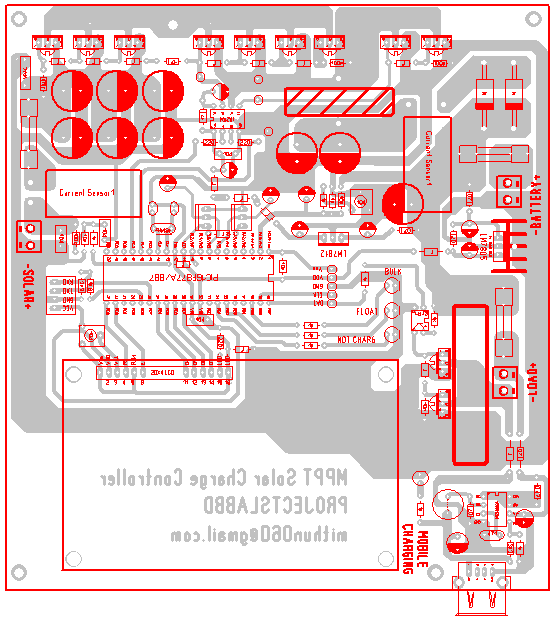PCB design

You can download the PCB file from here.

## Testing the project in real life:

I made the project for practical testing. Here is lab test result: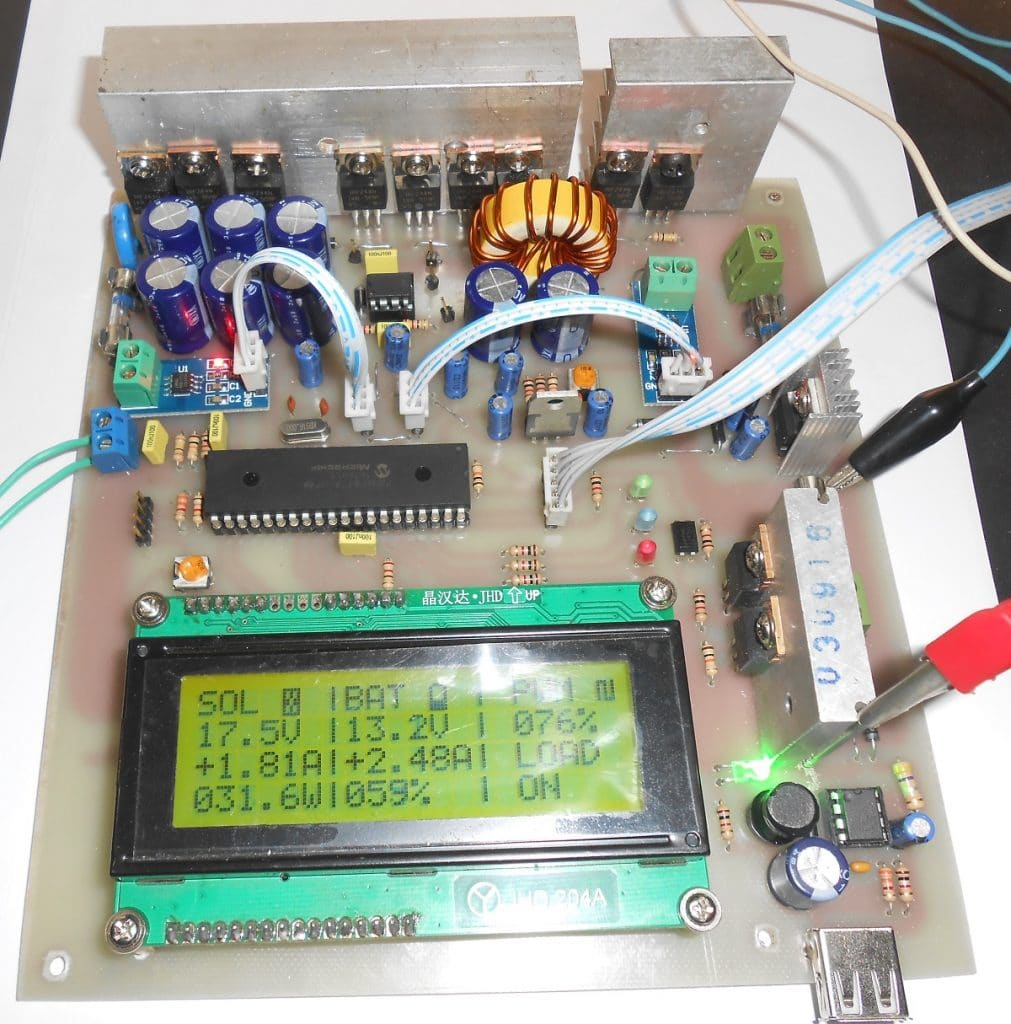MPPT Charge controller test 1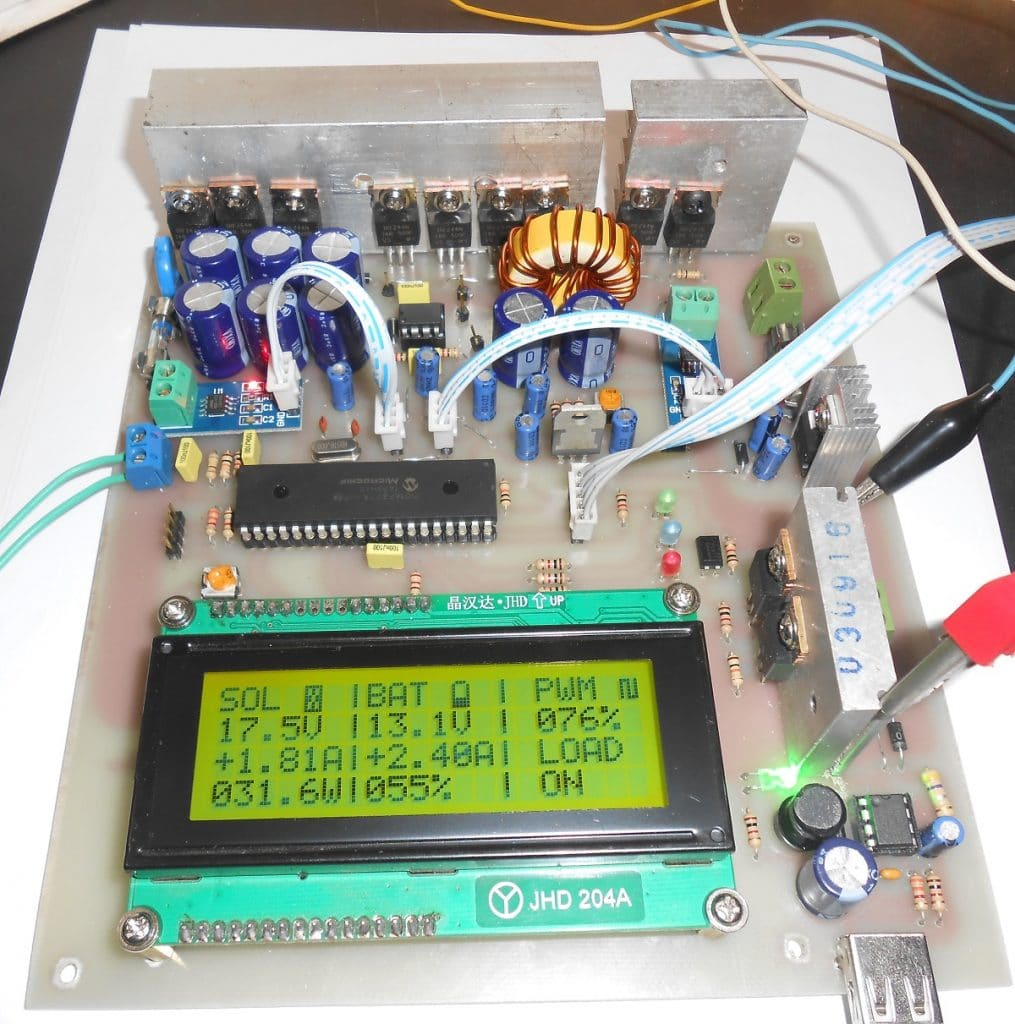MPPT Charge controller test 2

### About the field test result:

I gave this MPPT solar charge controller to one of my student who is being using this charge controller in his home solar power system. Hopefully the device is still running.

## Conclusion:

In the end, I hope you came this far. As it was a big project I omitted some theory parts what you should learn yourself. If I miss some important part, please let me know that. I’ll add that later on.

#### MKDas

Mithun K. Das; B.Sc. in EEE from KUET. Blog: https://labprojectsbd.com

### 6 Comments#### Sagheer Ahmad · January 25, 2021 at 4:28 pm

WILL THIS SOLAR Controller PCB board Operate charging at 24V volts. and I am a resident of Pakistan.
IN4917 is not available in Pakistan.what will be its replacement part?
Thank best Regard
Sagheer Ahmad#### Godwin · February 26, 2021 at 8:49 pm

Please,I need the professional type that can handle 12,24 and 48v solar panel and upto 40amp#### prabhash kumar · April 7, 2021 at 4:29 pm

Please share 24volt input DC to dc boost converter ..with code..gain good knowledge.. good job

#### Solar Charge Controller circuit & working principle of ON/OFF charge controller – Lab Projects BD · March 28, 2021 at 3:14 pm

[…] get the maximum power from a solar panel. I’ve posted an article on this. Please check this article here. I’ve explained detailed information about MPPT in that article. That is why I’m not […]